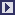## PCPACK6 Diagram Template Tool

 The Diagram Template Tool is used to create and edit diagram templates. Diagram templates are used to define the format and style of the diagrams created in the Diagram Tool. Any number of templates can be created and used for a knowledgebase. Diagram Templates Each diagram template determines the following: Which knowledge objects will be represented as nodes on the diagram The style (e.g. shape, colour, size) of each node type Which relations will be represented as links on the diagram The style (e.g. colour, end symbols, labels) of each type of link Which nodes will be allowed to be connected by which links Other diagram symbols (not represented in the knowledgebase) The operation of the Diagram Template Tool is very similar to the Diagram Tool. Basic Features Allows the creation of any network-style diagram format Any number of diagram templates Any number of nodes and links on each template Any nodes can be set so they can be decomposed (i.e. to show a 'lower level' diagram) Simple visual representation of the diagram template Special grid shows which relations are allowed between pairs of nodes Properties of nodes and links can be edited at any time (so will update existing diagrams) User Interface The user interface of the Diagram Template Tool is explained in the following figure.Other PCPACK tools:Protocol ToolLadder ToolDiagram ToolMatrix ToolAnnotation ToolAdmin ToolPublisher ToolEquation Editor ToolLauncher Wizard Tool